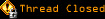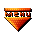# Topic awaiting preservation: site maps - regular expressions - counting slashes (Page 1 of 1)smonkey
Paranoid (IV) Inmate

From: Northumberland, England
Insane since: Apr 2003posted 05-14-2003 01:19

how can I divide/sort a php array in to multiple new arrays? there are some conditions to this so I will explain my situation:

I have a php script that recursively loops through all my website directories and gets all the htm/html/php files from them and put's them into an array as an absolute path. I would like to turn this array into some kind of file tree or easy to understand sitemap. I figure the array entries would need to be divided and sorted into a range of nested arrays or something and the sitemap made from that.
*My current locally tested array looks like :

 => c:\phpdev\www\ckg\splash.htm
 => c:\phpdev\www\ckg\index.htm
 => c:\phpdev\www\ckg\ckg\index.htm
 => c:\phpdev\www\ckg\ckg\thanks\index.htm
 => c:\phpdev\www\ckg\ckg\contact\index.htm
 => c:\phpdev\www\ckg\galleries\index.htm
 => c:\phpdev\www\ckg\galleries\submit\index.htm
 => c:\phpdev\www\ckg\galleries\artistlist\index.htm
 => c:\phpdev\www\ckg\galleries\photography\index.htm
 => c:\phpdev\www\ckg\galleries\media\index.htm
 => c:\phpdev\www\ckg\galleries\film\index.htm
 => c:\phpdev\www\ckg\galleries\art\index.htm
 => c:\phpdev\www\ckg\galleries\illustration\index.htm
 => c:\phpdev\www\ckg\galleries\illustration\thomas_meldgaard\index.htm
 => c:\phpdev\www\ckg\personal\jon\index.htm
 => c:\phpdev\www\ckg\personal\jay\index.htm
 => c:\phpdev\www\ckg\features\index.htm
 => c:\phpdev\www\ckg\features\hosting\index.htm
 => c:\phpdev\www\ckg\features\guestbook\index.htm
 => c:\phpdev\www\ckg\features\experiments\index.htm
 => c:\phpdev\www\ckg\features\experiments\text\text.htm
 => c:\phpdev\www\ckg\features\experiments\imgwall\imgwall1.htm
 => c:\phpdev\www\ckg\features\experiments\imgwall\imgwall2.htm
 => c:\phpdev\www\ckg\filelist.php
 => c:\phpdev\www\ckg\getfiles.php

Alternatively rather than make one array and then break it up into sub arrays is there a way that the following script can be made to put data into the nested arrays directly (each time it reads a sub directory in a directory create a new sub array etc.)? I basically want to run this script via a cron job every few days and create a seperate html sitemap file that is clear and understandable.<BLOCKQUOTE><FONT face="Verdana, Arial">code:</font><HR><pre><?php
$root_path = "."; function parseDir($path)
{
if (!($dir_handler = opendir($path))) {
die("Can't opendir() path '". $path ."'"); }$files = array();
while (($file = readdir($dir_handler)) !== false) {
$temp_path = realpath($path."/".$file); if ($file != "." && $file != "..") { if (is_dir($temp_path)) {
$files = array_merge($files, parseDir($temp_path)); } elseif (is_file($temp_path)) {
$pathInfo = pathinfo($temp_path);
if (preg_match("/(html?

Tyberius Prime
Paranoid (IV) Mad Scientist with Finglongers

From: Germany
Insane since: Sep 2001posted 05-14-2003 10:17

you can easily nest arrays.

just say
$shu = array(array(1,2),array(3,4)) then you can do things like$shu = 5;
or even $shu[] = 6; avidal Bipolar (III) Inmate From: austin, tx, usa Insane since: Nov 2000posted 05-24-2003 22:20 You can use variable variables also, I suppose. [code]$dir = "/test/";

$dir = str_replace("/","",$dir);

dir = "index.php";

echo \$test; //outputs "index.php" <--- Not tested, but I think that's how variable variables work.